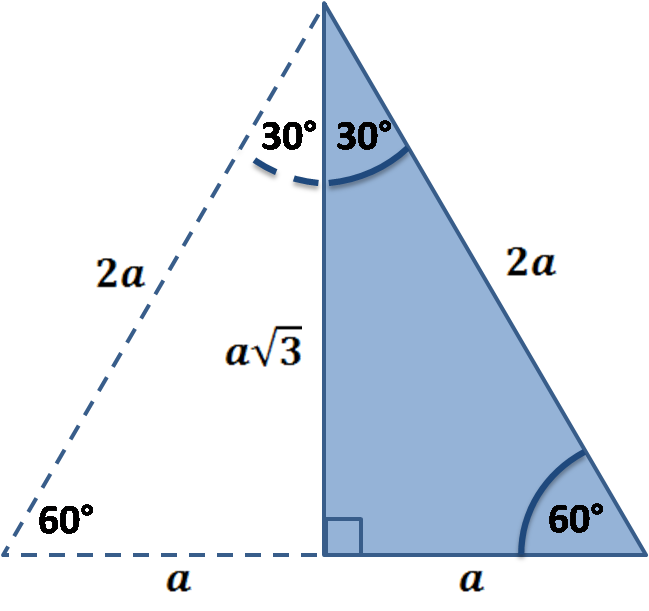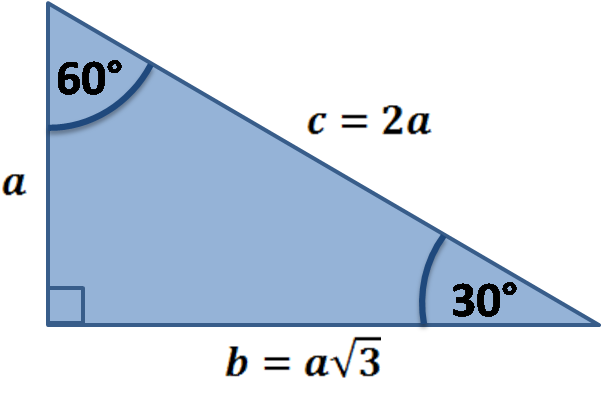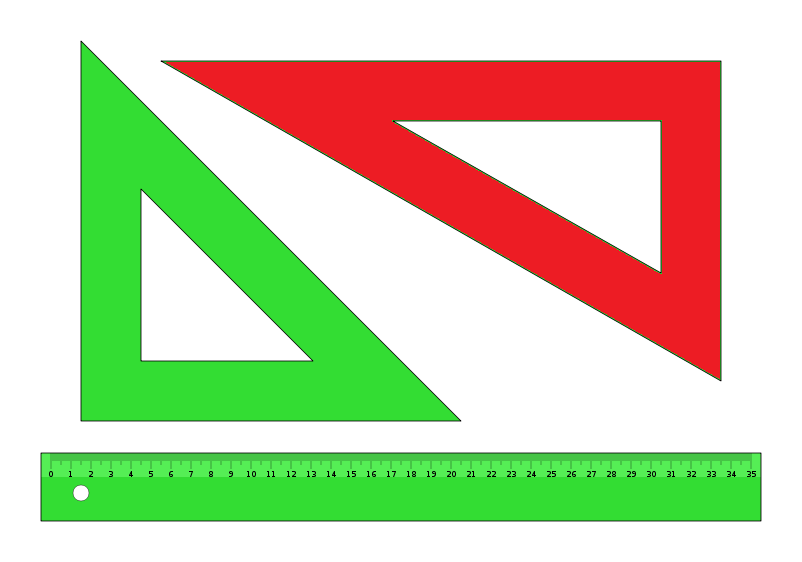# 30 60 90 Triangle Calculator

By Hanna Pamuła, PhD candidate
Last updated: Jun 26, 2020

With this 30 60 90 triangle calculator you can solve this special right triangle. Whether you're looking for the 30 60 90 triangle formulas for hypotenuse, wondering about 30 60 90 triangle ratio or simply you want to check how this triangle looks like, you've found the right website. Keep scrolling to learn more about this specific right triangle or check out our tool for the twin of our triangle - 45 45 90 triangle calc.

## How to solve a 30 60 90 triangle? 30 60 90 triangle formula

Assume that the shorter leg of a 30 60 90 triangle is equal to a. Then:

• the second leg is equal to a√3
• the hypotenuse is 2a
• the area is equal to a²√3/2
• the perimeter equals a(3 + √3)

The formulas are quite easy, but what's the math behind them? Let's check which methods you can use to prove them:1. Using the properties of the equilateral triangle

Did you notice that our triangle of interest is simply a half of the equilateral triangle? If you remember the formula for the height of such a regular triangle, you have the answer what's the second leg length. It's equal to side times a square root of 3, divided by 2:

`h = c√3/2`, `h = b` and `c = 2a` so `b = c√3/2 = a√3`

2. Using trigonometryIf you are familiar with the trigonometric basics, you can use, e.g. the sine and cosine of 30° to find out the others sides lengths:

`a/c = sin(30°) = 1/2` so `c = 2a`

`b/c = sin(60°) = √3/2` so `b = c√3/2 = a√3`

Also, if you know two sides of the triangle, you can find the third one from the Pythagorean theorem. However, the methods described above are more useful as they need to have only one side of the 30 60 90 triangle given.

## 30 60 90 triangle sides

If we know the shorter leg length `a`, we can find out that:

• `b = a√3`

• `c = 2a`

If the longer leg length `b` is the one parameter given, then:

• `a = b√3/3`

• `c = 2b√3/3`

For hypotenuse `c` known, the legs formulas look as follows:

• `a = c/2`

• `b = c√3/2`

Or simply type your given values and the 30 60 90 triangle calculator will do the rest!

## 30 60 90 triangle rules and properties

The most important rule to remember is that this special right triangle has one right angle and its sides are in an easy-to-remember consistent relationship with one another - the ratio is a : a√3 : 2a. Also, the unusual property of this 30 60 90 triangle is that it's the only right triangle with angles in an arithmetic progression.Triangles (set square). The red one is the 30-60-90 degree angle triangle

## 30 60 90 triangle ratioIn 30 60 90 triangle the ratios are:

• 1 : 2 : 3 for angles (30° : 60° : 90°)
• 1 : √3 : 2 for sides (a : a√3 : 2a)

## How to solve a 30 60 90 triangle - an example

You read about 30 60 90 triangle rules. Now it's high time you practiced!

1. Enter the given value. Let's say we want to check how to solve the 30 60 90 triangle from our triangle set. There's a scale on the longer leg, assume its length is 11 inches.
2. All the other values appear! Thanks to this 30 60 90 triangle calculator you find out that:
• shorter leg is 6.35 in - because `a = b√3/3 = 11in * √3/3 ~ 6.35 in`
• hypotenuse is equal to 12.7 in - because `c = 2b√3/3 = 2a ~ 12.7 in`
• area is 34.9 in² - it's the result of multiplying the legs length and dividing by 2 `area = a²√3 ≈ 34.9 in`
• perimeter equals 30.05 in - adding all sides gives that result `perimeter = a + a√3 + 2a = a(3 + √3) ≈ 30.05 in`
Hanna Pamuła, PhD candidatea
in
b
in
c
in
Area
in²
Perimeter
in
People also viewed…

### Car crash force

With this car crash calculator, you can find out how dangerous are car crashes.

### Humans vs vampires

Vampire apocalypse calculator shows what would happen if vampires were among us using the predator - prey model.

### Surface area of a triangular prism

The surface area of a triangular prism calculator is the simplest way to calculate the surface of many different types of triangular prisms.